# T-Hex 4DoF Leg Assembly Instructions

4 DOF T-HEX Leg Assembly Instructions.

Updated 11/11/2010

Safety first! Wear eye protection and never touch a powered robot!

Important! If you are building legs for the T-HEX robot, you will need to build three right legs (following the pictures on the right), and three left legs (by following the pictures on the left)! If you are building legs for your own creation, you can choose your own style.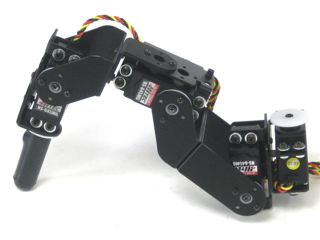Image of completed Right (robot's right) leg.

<>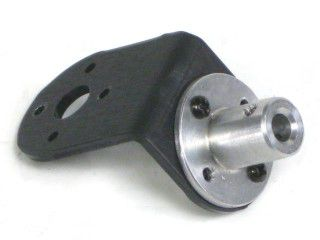Figure 1. (Left Leg)

Step 1.
Attach a Tubing Connector Hub to an "L" bracket as shown, using two 2-56 x .250 screws and 2-56 nuts. You will need to make six of these.

 12 x 12 x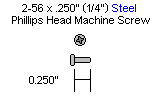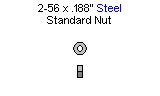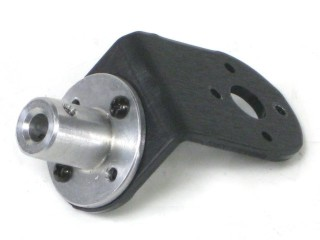Figure 1. (Right Leg)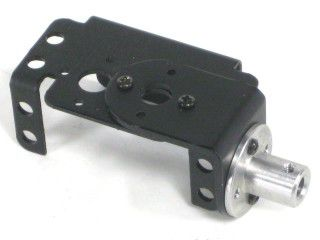Figure 2. (Left Leg)

Step 2.
Attach the other side of the "L" connector bracket to the Multi-Purpose bracket as shown, using two 2-56 x .250 screws and 2-56 nuts. Make three of each.

 12 x 12 x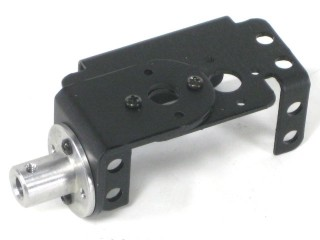Figure 2. (Right Leg)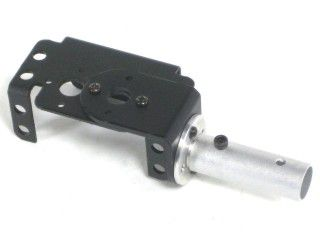Figure 3. (Left Leg)

Step 3.
Connect a 1.5" tube to each hub using a 4-40 x .250" screw.

 6 x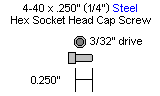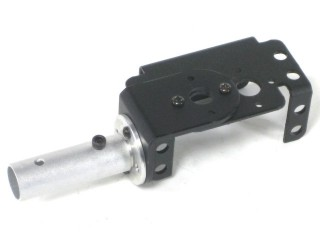Figure 3. (Right Leg)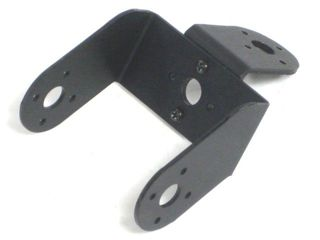Figure 4. (Left Leg)

Step 4.
Attach an offset "C" bracket to an "L" bracket as shown, using two 2-56 x .250 screws and 2-56 nuts. Create six of these.

 12 x 12 x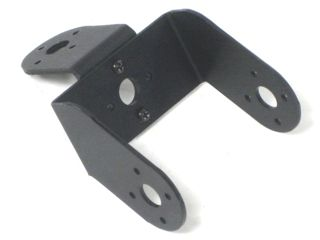Figure 4. (Right Leg)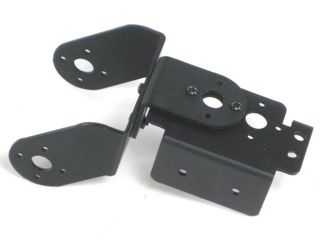Figure 5. (Left Leg)

Step 5.
Attach the other side of the six "L" connector brackets to a Multi-Purpose bracket as shown, using two 2-56 x .250 screws and 2-56 nuts. Make three for each side.

 12 x 12 x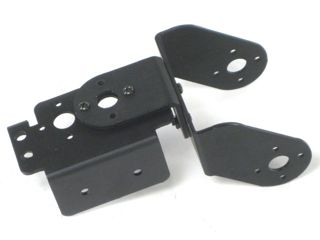Figure 5. (Right Leg)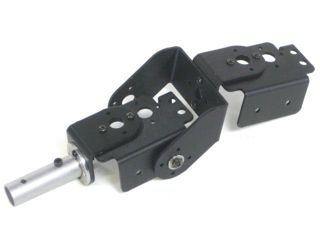Figure 6. (Left Leg)

Step 6.
Attach the offset "C" bracket to the Multi-Purpose bracket as shown. See the diagram below for detailed information. You should have three of each of these assemblies at the end of this step.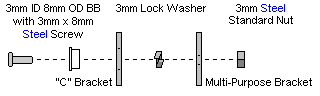Figure 6-1.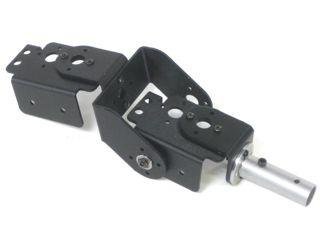Figure 6. (Right Leg)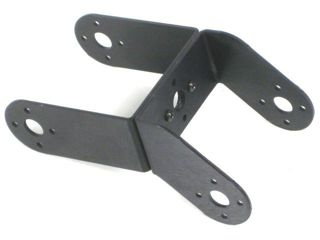Figure 7. (Left Leg)

Step 7.
Connect a long "C" bracket and an offset "C" bracket as shown, using two 2-56 x .250 screws and 2-56 nuts. Create six of these.

 12 x 12 x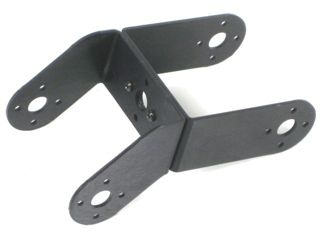Figure 7. (Right Leg)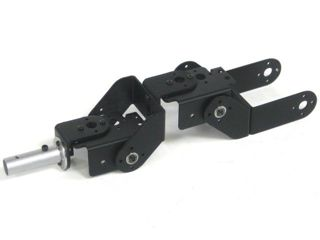Figure 8. (Left Leg)
Step 8.
Attach the "C" bracket to the Multi-Purpose bracket as shown. See the diagram below for detailed information. Make three of each.Figure 8-1.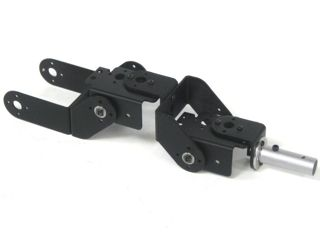Figure 8. (Right Leg)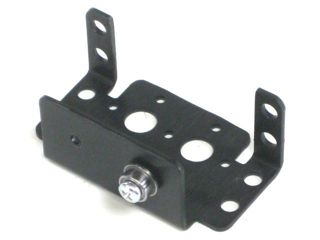Figure 9. (Left Leg)

Step 9.
Attach a ball bearing to the Multi-Purpose bracket as shown. See the diagram below for detailed information. Make three of each.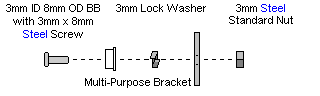Figure 9-1.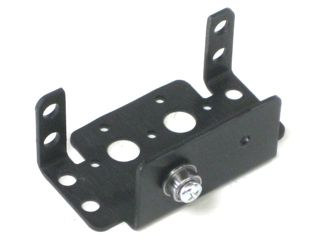Figure 9. (Right Leg)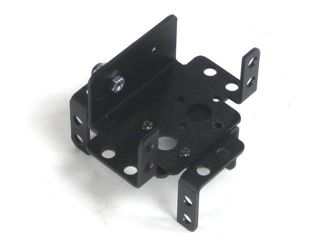Figure 10. (Left Leg)

Step 10. (Front and Middle Legs)
Attach two multi-purpose brackets as shown, using two 2-56 x .250" screws and 2-56 nuts. Make two for each side
.

 8 x 8 x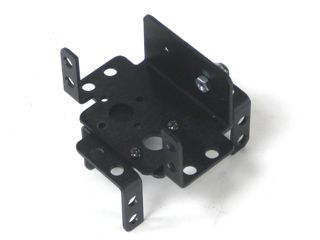Figure 10. (Right Leg)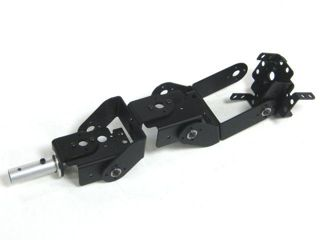Figure 11. (Left Leg)
Step 11. (Front and Middle Legs)
Attach the "C" bracket to the Multi-Purpose bracket as shown. See the diagram below for detailed information. Create two of each.Figure 11-1.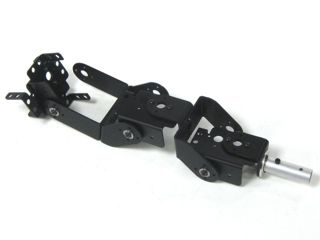Figure 11. (Right Leg)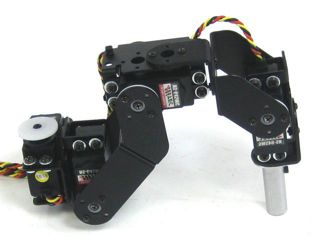Figure 12. (Left Leg)

Step 12. (Front and Middle Legs)
Install the servos as shown, using the included 3mm hardware, and #2 tapping screws.

 24 x 64 x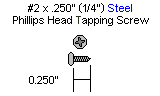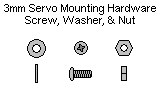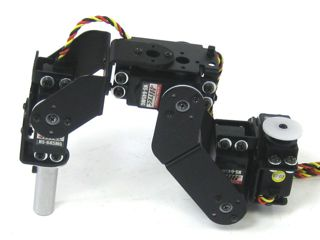Figure 12. (Right Leg)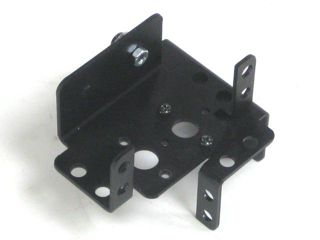Figure 13. (Left Leg)

Step 13. (Rear Legs)
Attach the two multi-purpose brackets as shown, using two 2-56 x .250" screws and 2-56 nuts. Make one for each side.

 4 x 4 x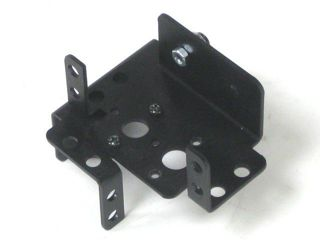Figure 13. (Right Leg)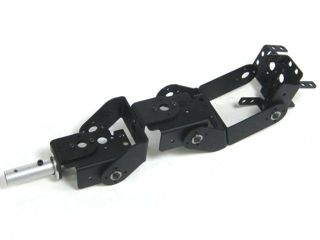Figure 14. (Left Leg)

Step 14. (Rear Legs)
Attach the "C" brackets to the Multi-Purpose bracket as shown. See the diagram below for detailed information.Figure 14-1.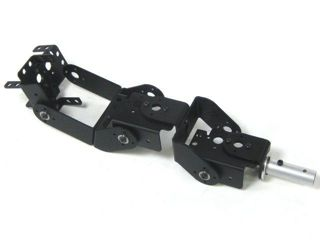Figure 14. (Right Leg)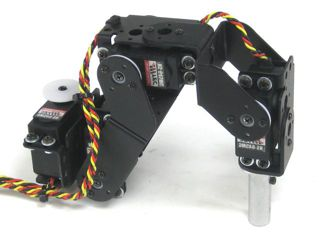Figure 15. (Left Leg)

Step 15. (Rear Legs)
Install the servos as shown, using the included 3mm hardware, and #2 tapping screws.

 12 x 32 x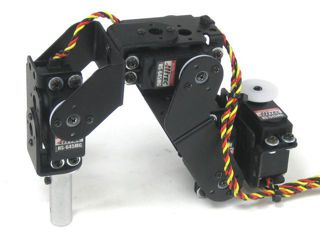Figure 15. (Right Leg)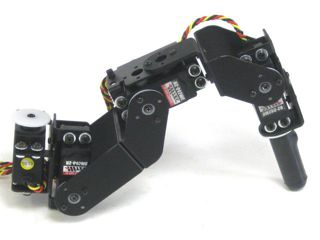Figure 16. (Left Leg)
Step 16. (All Legs)
Slide a rubber foot over the over the end of each 1.5" tube, and you're finished!Figure 16. (Right Leg)

Tags:
Created by Eric Nantel on 2023/01/26 13:49